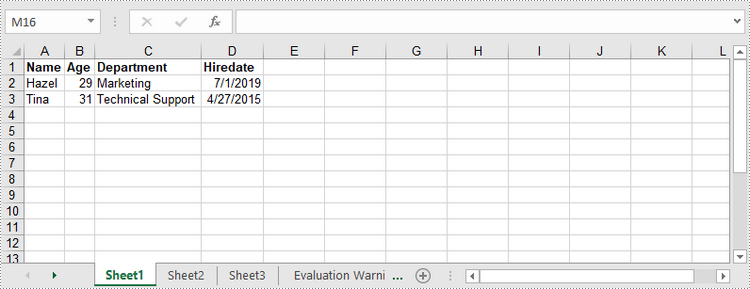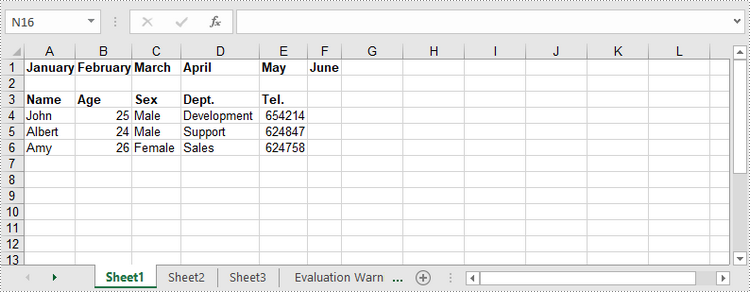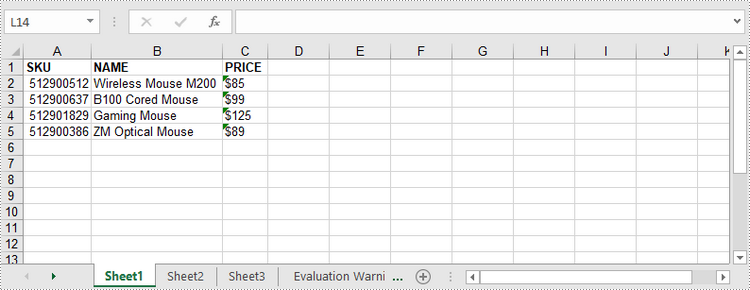# C#/VB.NET: Write Data to Excel Worksheets

Excel spreadsheets are scalable grid-based files that are used to organize data and perform calculations. People all across the world use spreadsheets to create tables for personal and business usage. To write a large amount of data into an Excel spreadsheet, it is recommended to use the programming method, which saves time and is less error-prone. In this article, you will learn how to write data into Excel in C# and VB.NET using Spire.XLS for .NET.

### Install Spire.XLS for .NET

To begin with, you need to add the DLL files included in the Spire.XLS for .NET package as references in your .NET project. The DLL files can be either downloaded from this link or installed via NuGet.

`PM> Install-Package Spire.XLS`

### Write Text or Number Values to Specific Cells

A certain cell in a worksheet can be accessed by Worksheet.Range[int row, int column] property. Then, you can add a text value or a number value to the cell through the XlsRange.Value or XlsRange.Value2 property. The following are the detailed steps.

• Create a Workbook object.
• Get the first worksheet through Workbook.Worksheets[] property.
• Get a specific cell through Workhseet.Range[] property.
• Add a text value or a number value to the cell through XlsRange.Value or XlsRange.Value2 property.
• Save the workbook to an Excel file using Workbook.SaveToFile() method.
• C#
• VB.NET
```using Spire.Xls;

namespace WriteDataToCells
{
class Program
{
static void Main(string[] args)
{
//Create a Workbook object
Workbook workbook = new Workbook();

//Get the first worksheet
Worksheet worksheet = workbook.Worksheets;

//Write data to specific cells
worksheet.Range[1, 1].Value = "Name";
worksheet.Range[1, 2].Value = "Age";
worksheet.Range[1, 3].Value = "Department";
worksheet.Range[1, 4].Value = "Hiredate";
worksheet.Range[1, 1].Value = "Hazel";
worksheet.Range[2, 2].Value2 = 29;
worksheet.Range[2, 3].Value = "Marketing";
worksheet.Range[2, 4].Value = "2019-07-01";
worksheet.Range[3, 1].Value = "Tina";
worksheet.Range[3, 2].Value2 = 31;
worksheet.Range[3, 3].Value = "Technical Support";
worksheet.Range[3, 4].Value = "2015-04-27";

//Auto fit column widths
worksheet.AllocatedRange.AutoFitColumns();

//Apply a style to the first row
style.Font.IsBold = true;
worksheet.Range[1, 1, 1, 4].Style = style;

//Save to an Excel file
workbook.SaveToFile("WriteToCells.xlsx", ExcelVersion.Version2016);
}
}
}```### Write Arrays to a Worksheet

Spire.XLS for Java provides the Worksheet.InsertArrary() method, allowing programmers to write one-dimensional arrays or two-dimensional arrays into the specified cell range of a worksheet. The steps to write arrays to a worksheet are as follows:

• Create a Workbook object.
• Get the first worksheet through Workbook.Worksheets[] property.
• Create a one-dimensional array and a two-dimensional array.
• Insert the arrays to worksheet using Worksheet.InsertArray() method.
• Save the workbook to an Excel file using Workbook.SaveToFile() method.
• C#
• VB.NET
```using Spire.Xls;

namespace WriteArraysToWorksheet
{
class Program
{
static void Main(string[] args)
{
//Create a Workbook instance
Workbook workbook = new Workbook();

//Get the first worksheet
Worksheet worksheet = workbook.Worksheets;

//Create a one-dimensional array
string[] oneDimensionalArray = new string[] { "January", "February", "March", "April", "May", "June" };

//Write the array to the first row of the worksheet
worksheet.InsertArray(oneDimensionalArray, 1, 1, false);

//Create a two-dimensional array
string[,] twoDimensionalArray = new string[,]{

{"Name", "Age", "Sex", "Dept.", "Tel."},
{"John", "25", "Male", "Development","654214"},
{"Albert", "24", "Male", "Support","624847"},
{"Amy", "26", "Female", "Sales","624758"}
};

//Write the array to the worksheet starting from the cell A3
worksheet.InsertArray(twoDimensionalArray, 3, 1);

//Auto fit column width in the located range
worksheet.AllocatedRange.AutoFitColumns();

//Apply a style to the first and the third row
style.Font.IsBold = true;
worksheet.Range[1, 1, 1, 6].Style = style;
worksheet.Range[3, 1, 3, 6].Style = style;

//Save to an Excel file
workbook.SaveToFile("InsertArrays.xlsx", ExcelVersion.Version2016);
}
}
}```### Write a DataTable to a Worksheet

To import data from a DataTable to a worksheet, use the Worksheet.InsertDataTable() method. The following are the detailed steps.

• Create a Workbook object.
• Get the first worksheet through Workbook.Worksheets[] property.
• Create a DataTable with random data.
• Write the DataTable to the worksheet at the specified location using Worksheet.InsertDataTable() method.
• Save the workbook to an Excel file using Workbook.SaveToFile() method.
• C#
• VB.NET
```using System;
using System.Data;
using Spire.Xls;

namespace WriteDataTableToWorksheet
{
class Program
{
static void Main(string[] args)
{
//Create a Workbook instance
Workbook workbook = new Workbook();

//Get the first worksheet
Worksheet worksheet = workbook.Worksheets;

//Create a DataTable object
DataTable dataTable = new DataTable();

DataRow dr = dataTable.NewRow();
dr = 512900512;
dr = "Wireless Mouse M200";
dr = "\$85";

dr = dataTable.NewRow();
dr = 512900637;
dr = "B100 Cored Mouse";
dr = "\$99";

dr = dataTable.NewRow();
dr = 512901829;
dr = "Gaming Mouse";
dr = "\$125";

dr = dataTable.NewRow();
dr = 512900386;
dr = "ZM Optical Mouse";
dr = "\$89";

//Write datatable to the worksheet
worksheet.InsertDataTable(dataTable, true, 1, 1, true);

//Auto fit column width in the located range
worksheet.AllocatedRange.AutoFitColumns();

//Apply a style to the first and the third row
style.Font.IsBold = true;
worksheet.Range[1, 1, 1, 3].Style = style;

//Save to an Excel file
workbook.SaveToFile("InsertDataTable.xlsx", ExcelVersion.Version2016);
}
}
}```### Apply for a Temporary License

If you'd like to remove the evaluation message from the generated documents, or to get rid of the function limitations, please request a 30-day trial license for yourself.